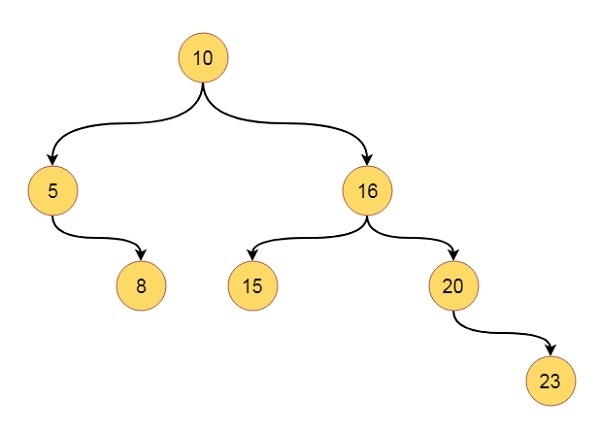# Preorder Tree Traversal in Data Structures

In this section we will see the pre-order traversal technique (recursive) for binary search tree.

Suppose we have one tree like this −The traversal sequence will be like: 10, 5, 8, 16, 15, 20, 23

## Algorithm

preorderTraverse(root):
Begin
if root is not empty, then
print the value of root
preorderTraversal(left of root)
preorderTraversal(right of root)
end if
End

## Example

Live Demo

#include<iostream>
using namespace std;
class node{
public:
int h_left, h_right, bf, value;
node *left, *right;
};
class tree{
private:
node *get_node(int key);
public:
node *root;
tree(){
root = NULL; //set root as NULL at the beginning
}
void preorder_traversal(node *r);
node *insert_node(node *root, int key);
};
node *tree::get_node(int key){
node *new_node;
new_node = new node; //create a new node dynamically
new_node->h_left = 0; new_node->h_right = 0;
new_node->bf = 0;
new_node->value = key; //store the value from given key
new_node->left = NULL; new_node->right = NULL;
return new_node;
}
void tree::preorder_traversal(node *r){
if(r != NULL){ //When root is present, visit left - root - right
cout << r->value << " ";
preorder_traversal(r->left);
preorder_traversal(r->right);
}
}
node *tree::insert_node(node *root, int key){
if(root == NULL){
return (get_node(key)); //when tree is empty, create a node as root
}
if(key < root->value){ //when key is smaller than root value, go to the left
root->left = insert_node(root->left, key);
}else if(key > root->value){ //when key is greater than root value, go to the right
root->right = insert_node(root->right, key);
}
return root; //when key is already present, do not insert it again
}
main(){
node *root;
tree my_tree;
//Insert some keys into the tree.
my_tree.root = my_tree.insert_node(my_tree.root, 10);
my_tree.root = my_tree.insert_node(my_tree.root, 5);
my_tree.root = my_tree.insert_node(my_tree.root, 16);
my_tree.root = my_tree.insert_node(my_tree.root, 20);
my_tree.root = my_tree.insert_node(my_tree.root, 15);
my_tree.root = my_tree.insert_node(my_tree.root, 8);
my_tree.root = my_tree.insert_node(my_tree.root, 23);
cout << "Pre-Order Traversal: ";
my_tree.preorder_traversal(my_tree.root);
}

## Output

Pre-Order Traversal: 10 5 8 16 15 20 23